# How to calculate quarter and year from date in Excel?

It's probable that if you run a company or pretty much any other area, you'll find yourself needing to know what calendar quarter a certain date comes under very often. Regrettably, Excel does not include a function that may produce this information depending on a specified date. We are able to transform any given date into a calendar quarter by using this method.

If you already have a column of dates and you want to achieve the following result, you will need to modify the dates to just include the quarter and the year.

## Step 1

Following is an easy formula that may assist you in calculating the quarter and year based on the supplied date. Please do so by following the instructions below.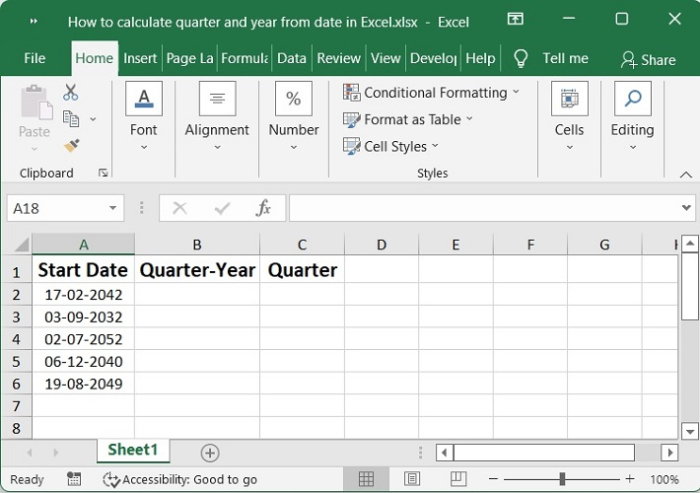## Step 2

After entering the following formula into a blank cell at the location where you wish to output the calculated result, the date will be presented in a manner that takes into account both the quarter and the year.

="Q" &INT((MONTH(A2)+2)/3) & "-" & YEAR(A2)


This snapshot displays the quarter in relation to the year.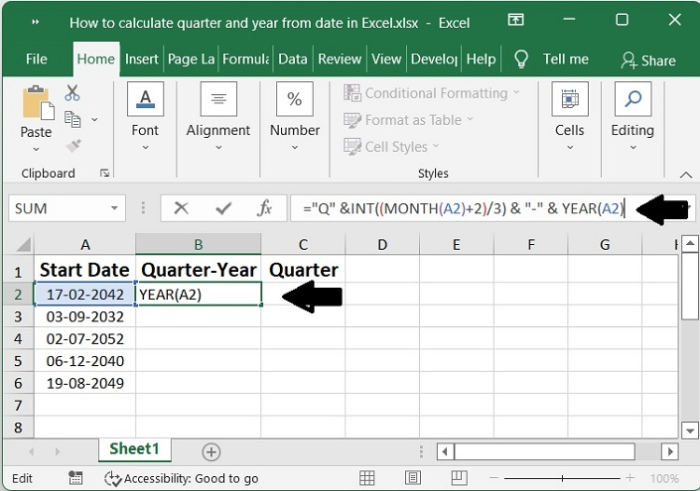## Step 3

In order to put this formula into action, you will need to drag the fill handle all the way down until it is touching the cells.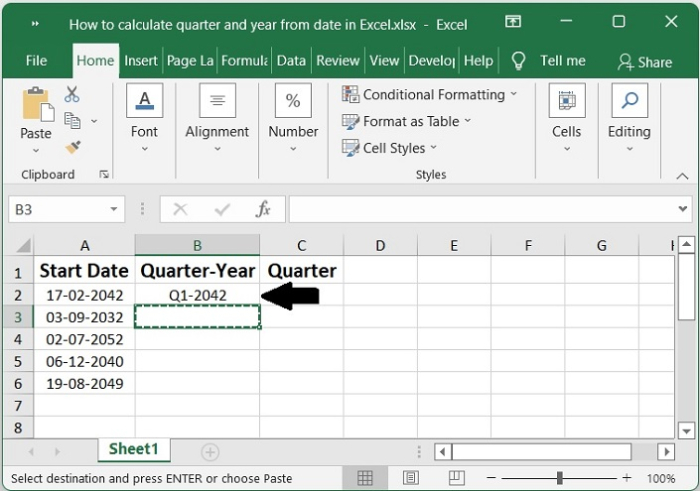## Step 4

If all you need to do is determine the quarter starting from the given date, please use the formula that is provided below.

="Q"&ROUNDUP(MONTH(A2)/3,0)


This snapshot gives an overview of the year without reference to the quarters.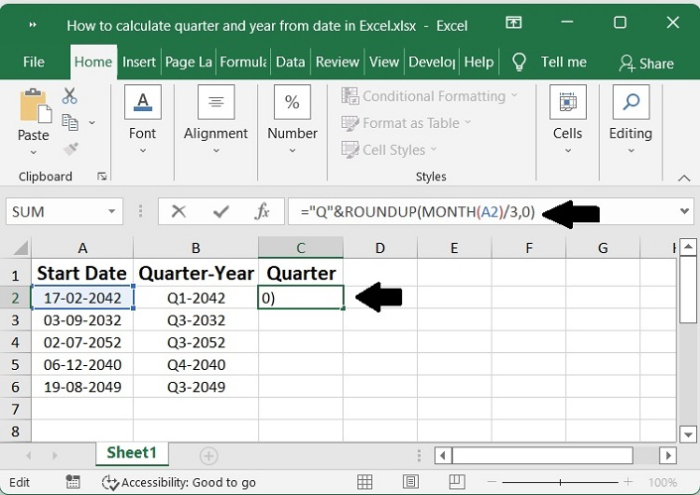## Step 5

After that, slide the fill handle down to the cells in order to fill in this formula. Note that only the quarter that corresponds to the current date is shown (for an example, see the screenshot).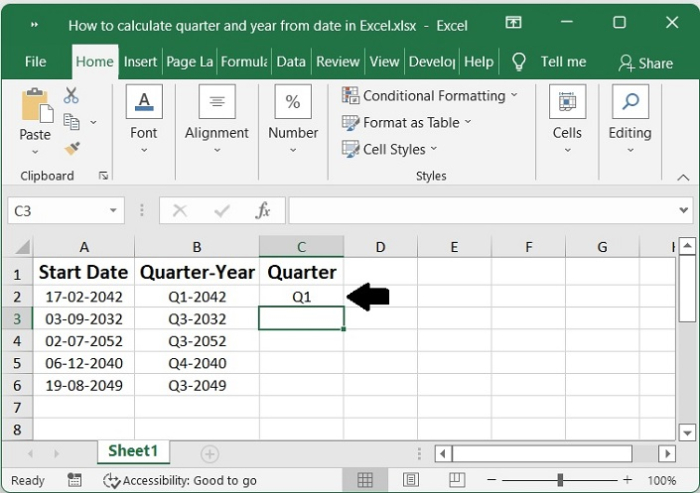## Step 6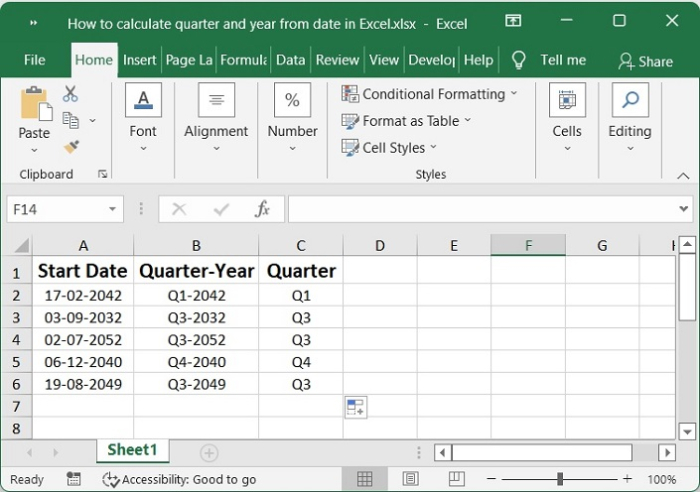## Conclusion

In this tutorial, we used a simple example to demonstrate how you can calculate quarter and year from a given date in Excel.

Updated on: 03-Feb-2023

1K+ Views